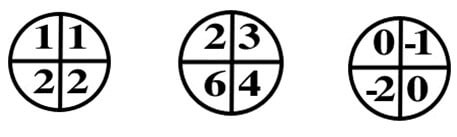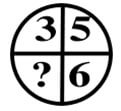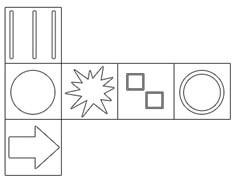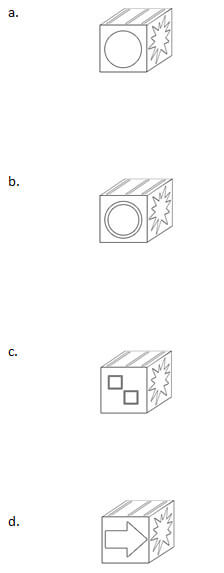# Wonderlic WPT-R Practice Test

##### 1. The numbers in the figures below have the same mathematical relationship to one another.##### What number should replace the question mark in the figure below?a 0
b 2
c 6
d 10

##### 2. Assume the first two statements are true. The final statement is:

a True
b False
c Not certain

1. All employees must submit time sheets weekly
2. Mike is an employee
3. Mike has submitted this week’s time sheet

##### 3. How many of the five pairs of items listed below are exact duplicates?

Bob Roberts | Rob Roberts
Shawn Phillips | Shaun Phillips
T.J. Paine | T.J. Paine
Tom Jensen | Tom Jansen
Pete Jacobs | Pete Jacobs

##### 4. RETRACT | RETRIEVE – these words:

a Have similar meanings
c Have neither similar nor contradictory meanings

##### 5. Observe the following shape:########### 1. D

10. The numbers in the bottom boxes are exactly twice the number diagonal to them in the top boxes. In the example, 1 X 2 = 2 in the first figure, 2 X 2 = 4 and 3 X 2 = 6 in the second figure, and 0 X 2 = 0 and -1 X 2 = -2 in the third figure. In the question, we see that 3 X 2 = 6, just as in the example, so we should multiply 5 by 2 to get the missing answer, which is 10.

###### 2. C

not certain. We know that Mike must submit a time sheet weekly, since he is an employee, but we do not know that he has submitted this week’s time sheet yet. It is possible, but not necessarily true, that he has done so already.

###### 3. T

are exact duplicates, T.J. Paine and Pete Jacobs. In the first pair, Bob and Rob do not match. In the second, Shawn and Shaun are spelled differently. In the fourth pair, Jensen and Jansen are spelled differently.

###### 4. A

have similar meanings. Both words involve taking something back; “to retract” something is to draw it back or withdraw it (for instance, a statement), and “to retrieve” something can mean to get it back or regain it.

###### 5. A

This is the only figure in which the three faces shown could appear in that orientation on a cube made from the two-dimensional shape given. In choice B, the two circles could be next to the other two figures, but the three stripes should be facing the other way. In choice C, the two squares should be on the side of the cube we cannot see, if we see the other two figures. In choice D, the arrow and the stripes could not be visible at the same time; they should be on opposite sides of the cube. Choice A is the only one that is logically possible.